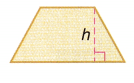Practice questions available in McGraw Hill Math Grade 6 Answer Key PDF Lesson 23.2 Quadrilaterals will engage students and is a great way of informal assessment.

Exercises

IDENTIFY

Determine what type of shape the figure is: square, rectangle, rhombus, trapezoid, or kite.

Question 1.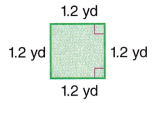The plane figure with four equal sides is called a square.

Question 2.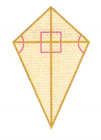The name of the above shape is kite

Question 3.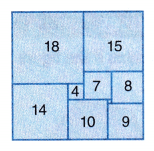18 + 14 = 32
18 + 15 = 33
14 + 10 + 9 = 33
15 + 8 + 9 = 32
The name of the above figure is rectangle.

Question 4.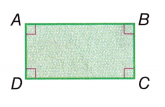A Rectangle is a four sided-polygon, having all the internal angles equal to 90 degrees.

Question 5.The trapezium is a flat shape or two-dimensional shape with 4 straight sides.

Question 6.The name of the shape is a rhombus with equal sides.

Question 7.